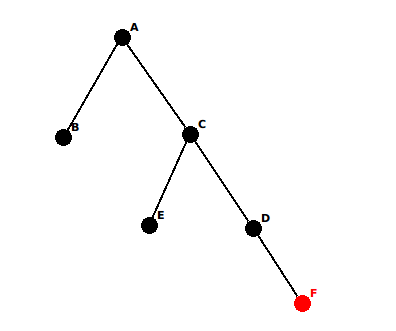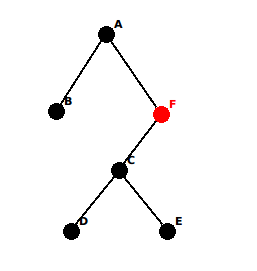## 热身题 - 24Game

### 题意

S = [1, 2, 3, 4, 5]

1 <= n <= 100000

### 解答

`f(5) => 24`略微复杂一点，但是也不在话下。这样我们的结论已经扩展到了`k >= 4`的所有整数。

## 归纳法

Let T be a theorem we want to prove.

Suppose that T includes a parameter n whose value can be any natural number.

Instead of proving directly that T holds for all values of n, we prove the following two conditions:

1. T holds for n = 1
2. For every n > 1, if T holds for n - 1, then T holds for n

## 归纳法应用 —— 笛卡尔树

### 换一种思考方式

1. `A[k+1] <= A[k]`，此时第k+1项会成为到第k项的右儿子，这样树展开时，第k项和第k+1项必然相联，并且也满足父节点必须比子节点大的条件。
2. `A[k+1] > A[k]`，第k+1项必然会成为第k项的左儿子。但是我们还需要考虑，第k项的父节点比第k+1项小的情况。经过思考，我们不难发现，第k+1项的左儿子t，一定是第k项祖先节点中小于第k+1项的最大值。而第k+1项则替换掉节点t的位置。```class Solution {
// ...
TreeNode* do_insert(int v) { // one node at a time
TreeNode* ptr = NULL;
TreeNode* now = new TreeNode(v);
while (!st.empty()) {
ptr = st.top();
if (ptr->value > v) {
now->left = ptr->right;
ptr->right=now;
st.push(now);
return root;
}
st.pop();
}
now->left = ptr;
st.push(now);
root = now;
return root;
}
// ...
};
```

## 课后习题 - 最大子段乘积

### 从零开始的想法

```class Solution { // a drunk moron who wrote this
public:
int maxProduct(int A[], int n) {
int p = 0, q = 0;
int ans = -INF;
int pro = 1;
for (int i = 0; i <= n; i++) {
if (i != n && A[i] != 0) {
pro *= A[i];
ans = max(pro, ans);
q = i + 1;
} else {
if (i < n && A[i] == 0) {
ans = max(ans, 0);
}
while (p < q) {
pro /= A[p];
p++;
if (p == q) {
break;
}
ans = max(pro, ans);
}
p = q = i + 1;
}
}
return ans;
}
private:
static const int INF = 0x3f3f3f3f;
};
```

### 用归纳法解决这个问题

```class Solution:
def maxProduct(self, A):
mini, maxi = (1, 1)